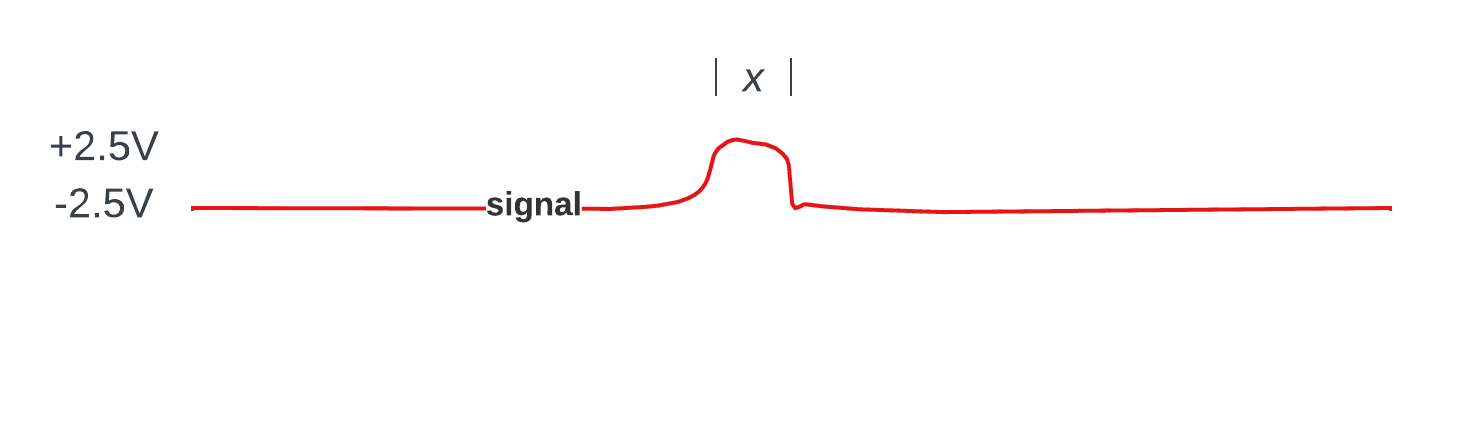a journal of interesting technical ideas . . .# Length of a bit

###### by J. D. Wegner on Monday, Aug 15, 2022

In the category of unexpected questions:

Over a glass of good whisky, a semi-technical friend of mine said, “Ok - I’ve been wondering about this, it’s appropos of nothing, but have no idea how to figure it out: How long (in length) is a bit?”

After thinking about it, I said it depends on a few factors, the most important of which is the frequency of the signal and the media that carries the signal.

“What does the media have to do with it, I thought electromagnetic signals traveled at the speed of light.”

I affirmed that they do - in a perfect vacuum. Wanna just go with that? “Sure, let’s start there.”

Since he was asking about the length of a single bit, we need a unit of distance; meters (m) works well here I think. We’ll start with a frequency of 1MHz.

The basic formula is $length = \frac{speed}{frequency}$ so …

$$length = \frac{300,000,000 m/s}{1,000,000 b/s} = 300 m/b$$

“That’s a lot longer than I thought it would be” he said.

You want it shorter? Increase the frequency of the signal. How about 1GHz? It’s just under a foot. $$length = \frac{300,000,000 m/s}{1,000,000,000 b/s} = 0.3 m/b$$

He said he got that, but what about the carrying medium?

“OK, light (and electromagnetic fields) travels about 300,000,000 m/sec in a vacuum. What about in a wire or fiber optic cable? I’d bet light travels slower there.”

Yup, you’re right. There is a velocity factor that is applicable to any conductor that carries a signal. That factor is sometimes expressed as a percentage of the speed of light in a vacuum. For example, in air the Velocity Factor (VF) is about 99%. In the typical coaxial cable used in cable TV it’s about 77%. For good ol’ twisted pair (Cat 6) the VF is 65%. The VF depends on the materials and the construction of the transmission line. Calculated by electrical engineers, the VF depends on the line’s tendency to impede the progress of the signal at various frequencies. That’s why each type of transmission line has a characteristic impedance which is related to the VF.

So, in space, a 1GHz “bit” is about a foot long. In a Cat 6 twisted pair cable it would be

$$length = \frac{300,000,000 m/s \times 0.65}{1,000,000,000/s} = 0.195 m/b$$

So as the signal goes through a higher impedance cable, the bits get shorter.

There are, of course, other factors to consider when engineering signal transmission systems in the real world. Very high- and low signaling rates need their own special considerations. One of the big advances in recent years is the ability to build smaller and more efficient integrated circuit (IC) chips that allow for faster and faster signal processing at lower power consumption. This is why we have supercomputers that can use so-called 5G frequencies and protocols - and also fit in your pocket. Sometimes we can even use them to make phone calls.